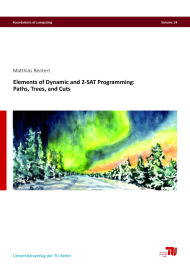# Elements of Dynamic and 2-SAT Programming: Paths, Trees, and CutsSize: 213 pages
Format: 14,8 x 21,0 cm
Publishing year: 2021
ISBN 978-3-7983-3209-6
12,00
This thesis presents faster (in terms of worst-case running times) exact algorithms for special cases of graph problems through dynamic programming and 2-SAT programming. Dynamic programming describes the procedure of breaking down a problem recursively into overlapping subproblems, that is, subproblems with common subsubproblems. Given optimal solutions to these subproblems, the dynamic program then combines them into an optimal solution for the original problem. 2-SAT programming refers to the procedure of reducing a problem to a set of 2-SAT formulas, that is, boolean formulas in conjunctive normal form in which each clause contains at most two literals.
Computing whether such a formula is satisfiable (and computing a satisfying truth assignment, if one exists) takes linear time in the formula length. Hence, when satisfying truth assignments to some 2-SAT formulas correspond to a solution of the original problem and all formulas can be computed efficiently, that is, in polynomial time in the input size of the original problem, then the original problem can be solved in polynomial time. We next describe our main results.
Diameter asks for the maximal distance between any two vertices in a given undirected graph. It is arguably among the most fundamental graph parameters. We provide both positive and negative parameterized results for distance-from-triviality-type parameters and parameter combinations that were observed to be small in real-world applications.
In Length-Bounded Cut, we search for a bounded-size set of edges that intersects all paths between two given vertices of at most some given length. We confirm a conjecture from the literature by providing a polynomial-time algorithm for proper interval graphs which is based on dynamic programming.
k-Disjoint Shortest Paths is the problem of finding (vertex-)disjoint paths between given vertex terminals such that each of these paths is a shortest path between the respective terminals. Its complexity for constant k > 2 has been an open problem for over 20 years. Using dynamic programming, we show that k-Disjoint Shortest Paths can be solved in polynomial time for each constant k.
The problem Tree Containment asks whether a phylogenetic tree T is contained in a phylogenetic network N. A phylogenetic network (or tree) is a leaf-labeled single-source directed acyclic graph (or tree) in which each vertex has in-degree at most one or out-degree at most one. The problem stems from computational biology in the context of the tree of life (the history of speciation). We introduce a particular variant that resembles certain types of uncertainty in the input. We show that if each leaf label occurs at most twice in a phylogenetic tree N, then the problem can be solved in polynomial time and if labels can occur up to three times, then the problem becomes NP-hard.
Lastly, Reachable Object is the problem of deciding whether there is a sequence of rational trades of objects among agents such that a given agent can obtain a certain object. A rational trade is a swap of objects between two agents where both agents profit from the swap, that is, they receive objects they prefer over the objects they trade away. This problem can be seen as a natural generalization of the well-known and well-studied Housing Market problem where the agents are arranged in a graph and only neighboring agents can trade objects. We prove a dichotomy result that states that the problem is polynomial-time solvable if each agent prefers at most two objects over its initially held object and it is NP-hard if each agent prefers at most three objects over its initially held object. We also provide a polynomial-time 2-SAT program for the case where the graph of agents is a cycle.